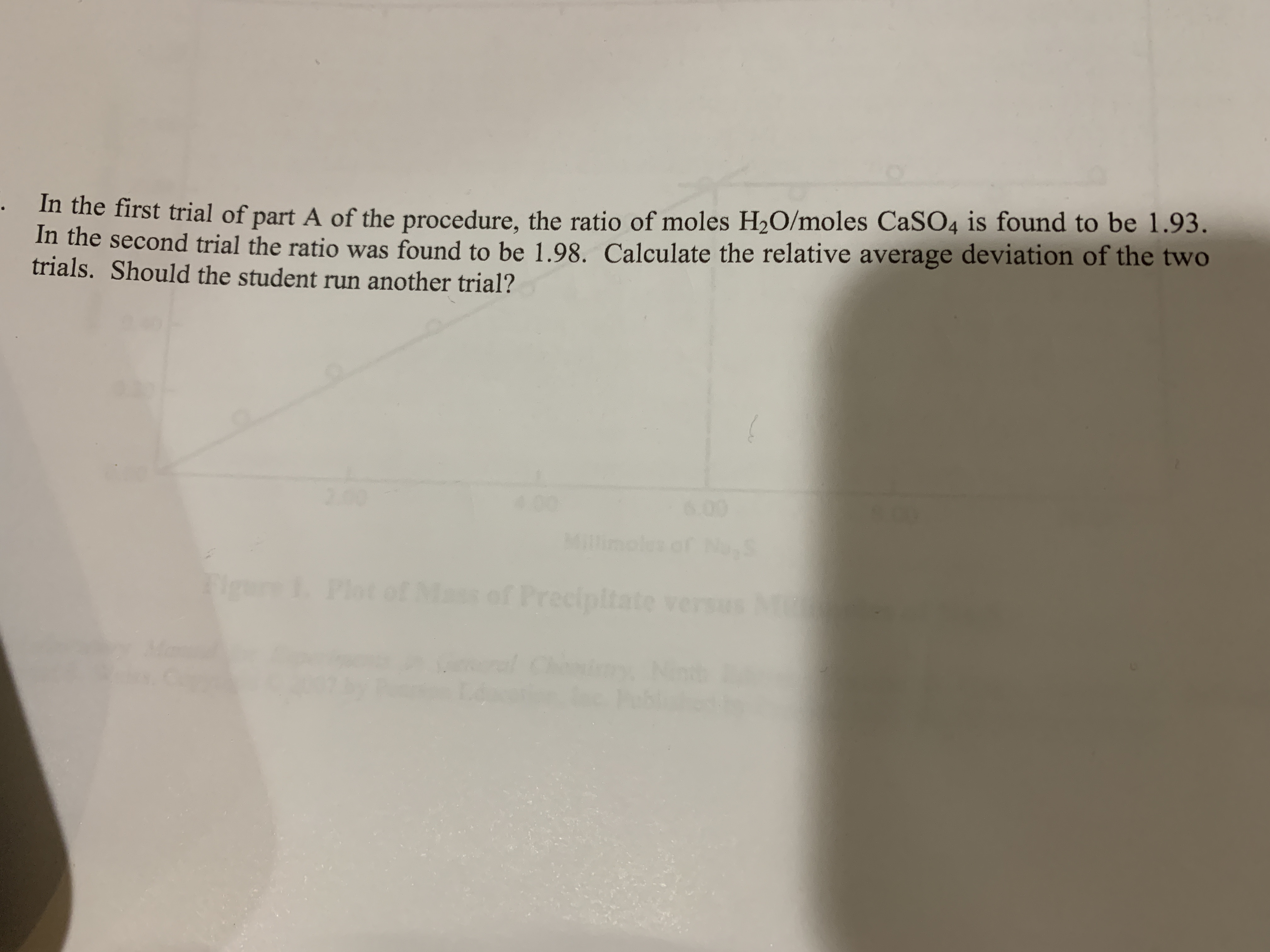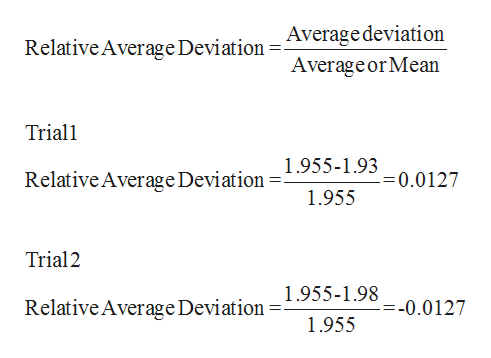In the first trial of part A of the procedure, the ratio of moles H2O/moles CaSO4 is found to be 1.93.In the second trial the ratio was found to be 1.98. Calculate the relative average deviation of the twotrials. Should the student run another trial?2004 06.09els ofesPlot of Mass of Precipitate

Question

Calculate the relative average deviation of the two trials. Should the student run another trial?help_outlineImage TranscriptioncloseIn the first trial of part A of the procedure, the ratio of moles H2O/moles CaSO4 is found to be 1.93. In the second trial the ratio was found to be 1.98. Calculate the relative average deviation of the two trials. Should the student run another trial? 200 4 0 6.09 els of es Plot of Mass of Precipitate fullscreen
Step 1

Average of two trials is given below:

Step 2

Relative average deviation can be dete...help_outlineImage TranscriptioncloseRelative Average Deviation = Average deviation Averageor Mean Trial1 Relative Average Deviation1.955-1.93 1.955 =0.0127 Trial2 Relative Average Deviation =1.955-1.98 1.955 =-0.0127 fullscreen

Want to see the full answer?

See Solution

Want to see this answer and more?

Our solutions are written by experts, many with advanced degrees, and available 24/7

See Solution
Tagged in

General Chemistry